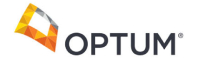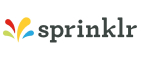New update is available. Click here to update.
Topics

# Closest Sum

Moderate0/80
Average time to solve is 30m+7 more companies

## Problem statement

Given an array 'ARR'' of 'N' integers and an integer 'target', your task is to find three integers in 'ARR' such that the sum is closest to the target.

Note
``````In the case of two closest sums, print the smallest sum.
``````
Detailed explanation ( Input/output format, Notes, Images )
Constraints
``````1 <= T <= 10
3 <= N <= 100
-10^5 <= Arr[i] <= 10^5
-3 * 10^5 <= target <= 3 * 10^5

where Arr[i] refers to the elements of the array.

Time Limit: 1 sec
``````
Sample Input 1
``````2
4
-1 2 1 -4
1
5
1 2 3 4 -5
10
``````
Sample Output 1
``````2
9
``````
Explanation of Sample Input 1
``````Test Case 1:
Sum of triplets:
(-1) + 2 + 1 = 2
(-1) + 2 + (-4) = -3
2 + 1 + (-4) = -1
(-1) + 1 + (-4) = -4
Out of all the triplet sums, 2 is closest to 1.

Test Case 2: Sum of triplet {2, 3, 4 } i.e. 9 is the closest sum to 10.
``````
Sample Output 2
``````2
5
10 12 7 8 -5
16
4
6 8 2 5
20
``````
Sample Output 2
``````15
19
``````Console